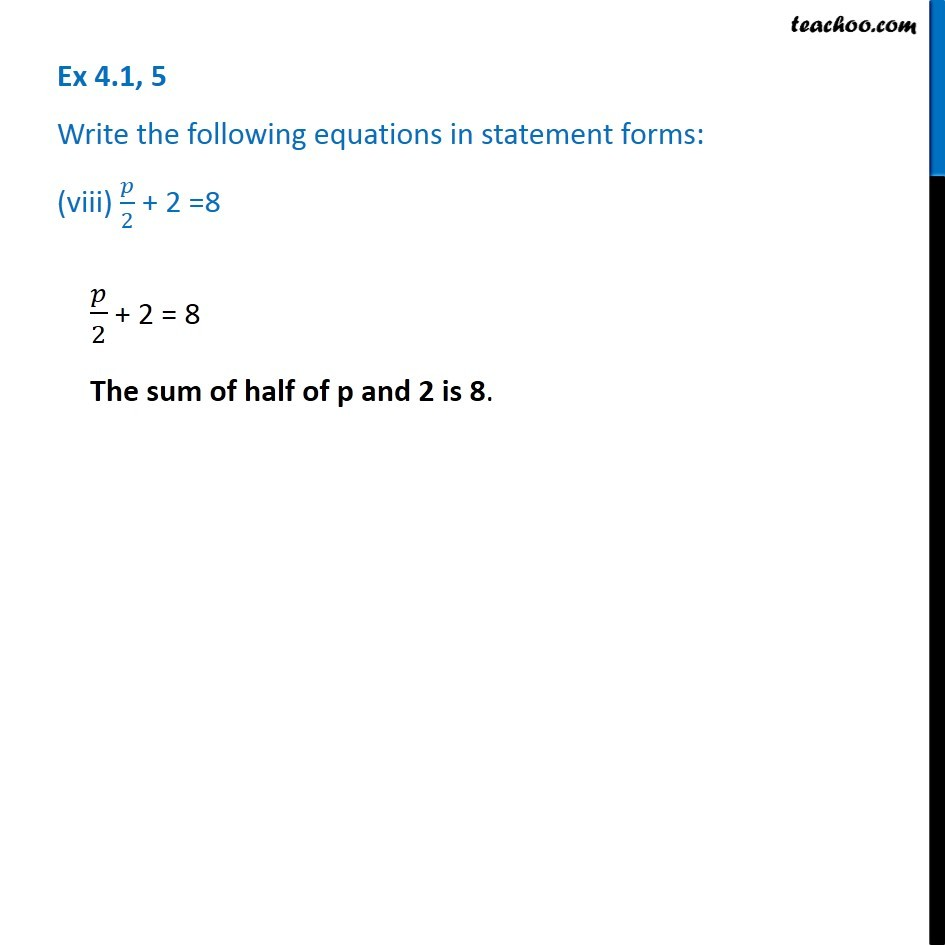1. Chapter 4 Class 7 Simple Equations
2. Serial order wise
3. Ex 4.1

Transcript

Ex 4.1, 5 Write the following equations in statement forms: (viii) 𝑝/2 + 2 =8 𝑝/2 + 2 = 8 The sum of half of p and 2 is 8.